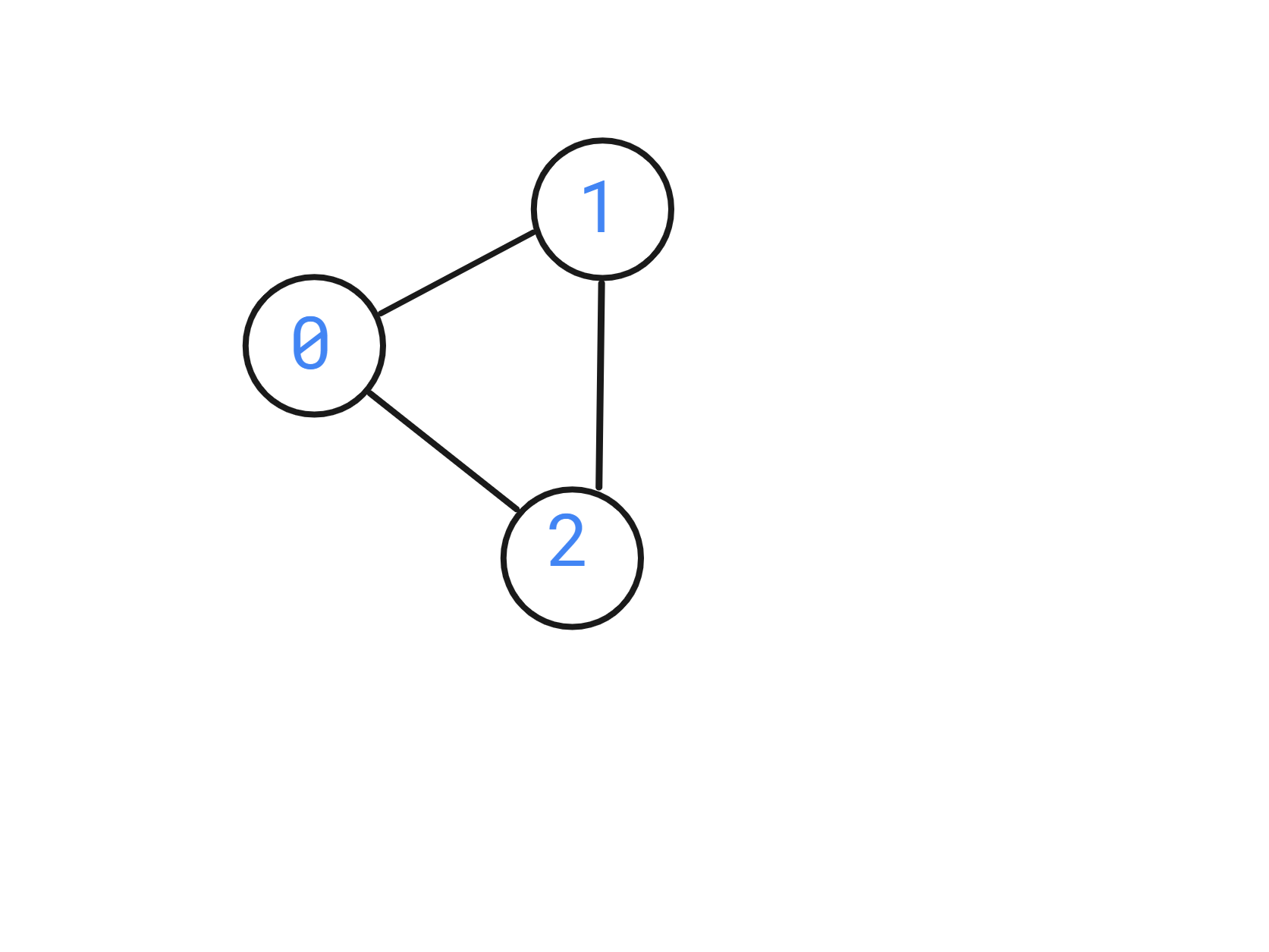# Bipartite Graph

Posted: 7 Dec, 2020
Difficulty: Moderate

## PROBLEM STATEMENT

#### You are given a 2D array ‘edges’ which contains 0 and 1, where ‘edges[i][j]’ = 1 denotes a bi-directional edge from ‘i’ to ‘j’.

##### Note:
``````If edges[i][j] = 1, that implies there is a bi-directional edge between ‘i’ and ‘j’, that means there exists both edges from ‘i’ to ‘j’ and to ‘j’ to ‘i’.
``````

#### For example

``````Given:
‘N’ = 3
‘edges’ = [[0, 1, 1], [0, 0, 1], [0,0,0]].
``````##### Input format:
``````The first line of input contains an integer ‘T’ denoting the number of test cases.

The first line of each test case contains two space-separated integers, ‘N,’ where ‘N’ is the number of rows in ‘edges’ and the number of columns in ‘edges’.

The next ‘N’ line of each test case contains ‘N’ space-separated integers which tell if there is an edge between ‘i’ and ‘j’.
``````
##### Output Format :
``````For each test case, You are supposed to return a bool value determining whether the graph is bipartite or not.
``````
##### Note:
``````You are not required to print the expected output; it has already been taken care of. Just implement the function.
``````
##### Constraints:
``````1 <= ‘T’ <= 10
2 <= ‘N’ <= 300
0 <= ‘edges[i][j]’ <= 1.

Time Limit: 1sec.
``````Approach 1

The idea is that a bipartite can be colored in such a way that only 2 colors in total would be used,  where the neighbor nodes have different colors. Therefore we can do a breadth-first search and assign colors to each vertex and if at any point neighboring nodes have the same color we return false.

The steps are as follows:

• Parse the given ‘edges’ into an adjacency matrix ‘graph’, by pushing ‘i’ is graph[j] and ‘j’ in graph[i] if edges[i][j] is 1.
• Maintain a vector ‘color’ which denotes the color of the ‘i’ the node, initially, all colors are un-assigned, hence -1.
• Maintain a color ‘c’ initially 0 to assign to nodes and flip after each level of the graph.
• Now maintain a queue ‘que’ for doing a breadth-first traversal and push ‘i’, the root node in it.
• While ‘que’ is not empty:
• Maintain a variable ‘node’ which denotes the node in the front of the ‘que’.
• Traverse all the neighbors ‘nbr’ of the current node.
• If the ‘color[nbr]’ is equal to ‘color[node]’, return false, since this is not possible, as discussed above.
• Else if the ‘color[nbr]’ is -1, which means it is unassigned, assign it ‘c’.
• Flip the color after traversing all the ‘nbr’ of ‘node’.
• If we exit the loop, return true, since all nodes have been assigned colors and no 2 adjacent nodes have the same color.